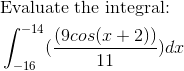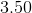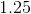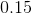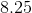AP Calculus AB : Antiderivatives following directly from derivatives of basic functions

Example Questions

← Previous 1 3 4 5

Example Question #2 : Calculus I — Derivatives

Consider the function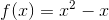Find the minimum of the function on the interval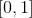.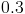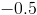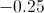Explanation:

To find potential minima of the function, take the first derivative ofusing the power rule.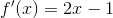Set the derivative to 0: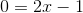We solve forto obtain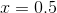and then plug in 0.5 into the original function to obtain the answer of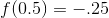We can double check thatis indeed a minimum by using the second derivative test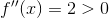which means the function is concave up, so that the point we found is a minimum.

Example Question #621 : Ap Calculus Ab

Find the x coordinate of the minimum of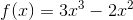on the interval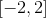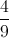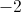Explanation:

First, find the derivative, which is: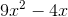Set that equal to 0 to find your critical points: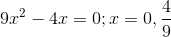Evaluate f at each critical point and endpoint over [-2, 2]: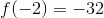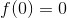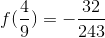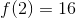The least of those numbers is the minimum. Because f(-2)=-32, that is the minimum.

Example Question #6 : Calculus I — Derivatives

What is the local minimum of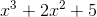when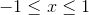?

The y-value is constant throughout that range.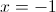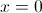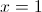There is no local minimum in that range.Explanation:

To find the maximum, we need to look at the first derivative.

To find the first derivative, we can use the power rule. To do that, we lower the exponent on the variables by one and multiply by the original exponent.

We're going to treatas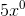since anything to the zero power is one.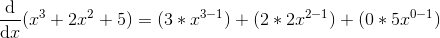Notice that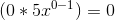since anything times zero is zero.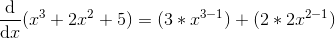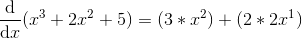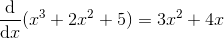When looking at the first derivative, remember that if the output of this equation is positive, the original function is increasing. If the derivative is negative, then the function is decreasing.

Because we want the MINIMUM, we want to see where the derivative changes from negative to positive.

Notice that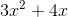has a root when. In fact, it changes from negative to positive at that particular point. This is the local minimum in the interval.

Example Question #622 : Ap Calculus Ab

Integrate,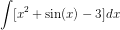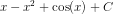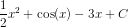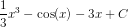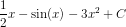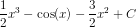Explanation:

Integrate1) Apply the sum rule for integration,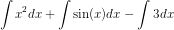2) Integrate each individual term and include a constant of integration,Further Discussion

Since indefinite integration is essentially a reverse process of differentiation, check your result by computing its' derivative.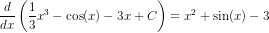This is the same function we integrated, which confirms our result. Also, because the derivative of a constant is always zero, we must include "C" in our result since any constant added to any function will produce the same derivative.

Example Question #623 : Ap Calculus Ab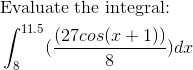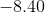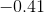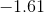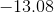Explanation: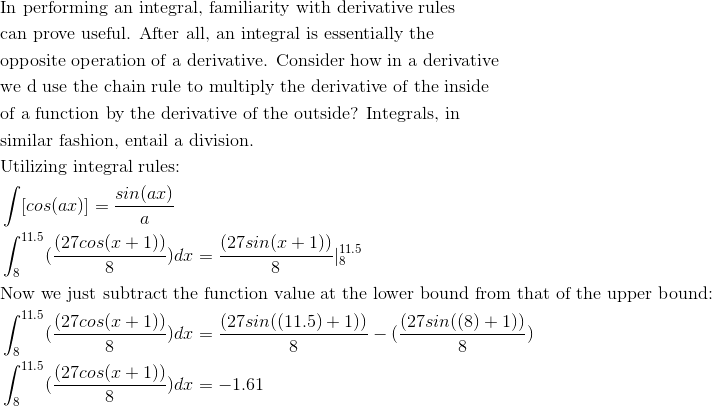Example Question #624 : Ap Calculus Ab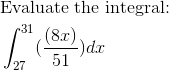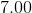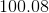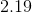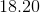Explanation: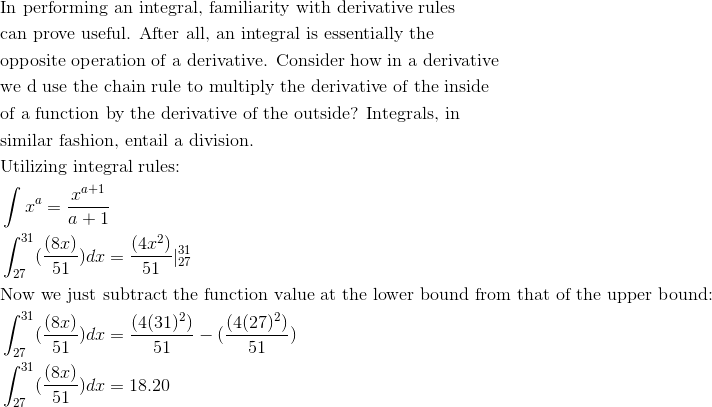Example Question #625 : Ap Calculus Ab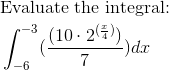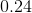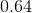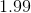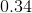Explanation: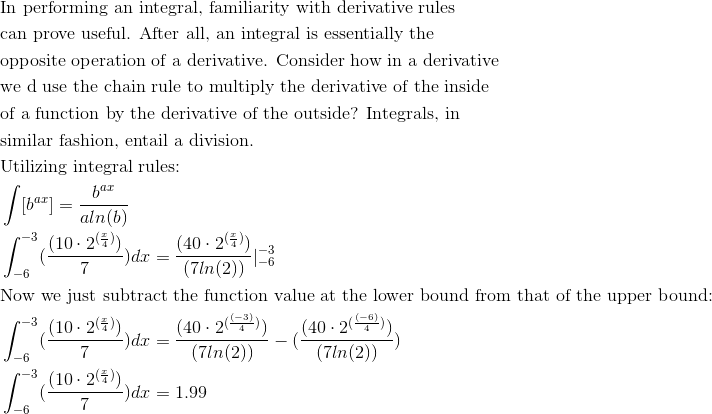Example Question #1 : Antiderivatives Following Directly From Derivatives Of Basic Functions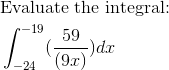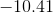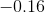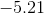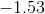Explanation: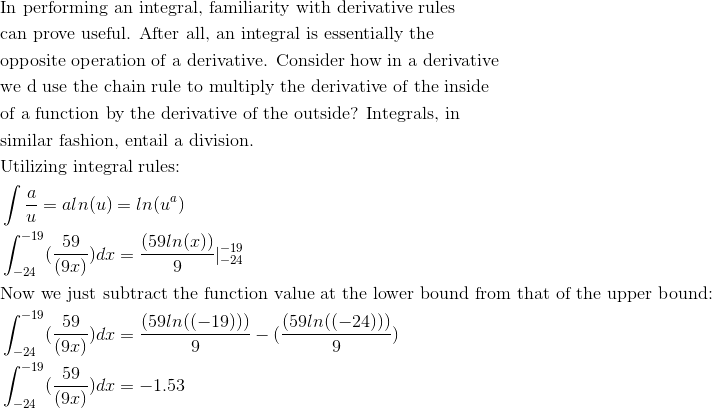Example Question #2 : Antiderivatives Following Directly From Derivatives Of Basic Functions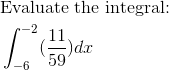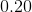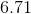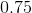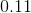Explanation: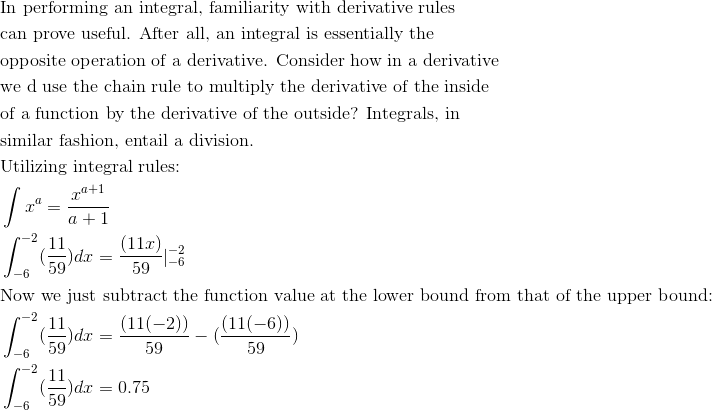Example Question #3 : Antiderivatives Following Directly From Derivatives Of Basic Functions